U.S. Department of Transportation
1200 New Jersey Avenue, SE
Washington, DC 20590
202-366-4000

Federal Highway Administration Research and Technology
Coordinating, Developing, and Delivering Highway Transportation Innovations

 REPORT This report is an archived publication and may contain dated technical, contact, and link information
 Federal Highway Administration > Publications > Research Publications > 14081 > 003.Cfm > Chapter 2
 Publication Number:  FHWA-HRT-14-081    Date:  November 2014
 Publication Number: FHWA-HRT-14-081 Date: November 2014

# Enhancing Statistical Methodologies For Highway Safety Research – Impetus From FHWA

## CHAPTER 2. OPPORTUNITIES FOR NEW OR ENHANCED METHODOLOGIES EMERGING FROM THE TECHNICAL EXPERTS MEETING

This section provides summary information on the most promising and relevant tools and methodologies that emerged from the technical experts meeting presentations and discussions. Eight such items were identified. Some of these items entail relatively technical methodology, and it is beyond the scope of this document to describe those in full detail. However, interested readers can easily learn more by examining standard texts in statistics, or by searching on keywords to find pertinent research papers.

### The Cox Proportional Hazards Model

The advantage of the Cox proportional hazards model is that it has been extensively studied and elaborated upon in the statistical research community, usually in the context of medical applications (e.g., How does weight, alcohol consumption and smoking affect the risk of heart attack at age 60?). In particular, there are methods for handling time-varying covariates, assessing goodness of fit, and setting confidence intervals, as well as addressing much more complex interactions. For example, in highway studies, illumination and traffic volume both vary by time of day. Below is an outline on how the model might be applied in highway safety analysis.

Suppose Y is a random variable denoting the time until the next automobile crash on a given short stretch of roadway, where the roadway has various characteristics X1, …, Xp, where those characteristics are explanatory variables such as speed limit, illumination, number of lanes, and so forth. If Y has the density function f(y), then its hazard function is defined as f(y)/[1 - F(y)], where F(y) is the cumulative distribution function of Y. The hazard function can be interpreted as the probability of a crash in the next instant, given that there have been no crashes in the previous y time units.

The Cox proportional hazards model is a key tool for studying hazard functions. The model form is as follows: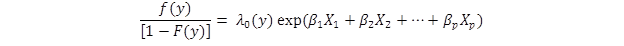Figure 3. Equation. Cox proportional hazards model.

Where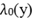is a baseline hazard function and the exponential term shows how roadway characteristics elevate or reduce the risk of a crash.

Specifically, if there are two factors, where X1 is road curvature and X2 is shoulder width, then the CMF for X1 is exp(β1X1), since this is the multiplier that shows how a specific value for curvature increases or decreases the crash risk compared to the baseline risk.

This model, which has had some application in other areas of safety research, is clearly relevant for CMF estimation. As a caution in using it for this purpose, goodness of fit concerns arise if the form of the model does not correctly describe the data, perhaps because some key variable or interaction has not been included, or because some transformation is required. And principled confidence intervals on the βj terms allow one to decide whether or not a specific characteristic is significantly relevant to the hazard function.

### Non-parametric Regressions

Classical regression assumes there is an additive linear relationship between the mean response and the predictor variables, as follows: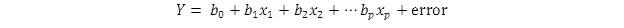Figure 4. Equation. Classic linear regression model.

(In developing SPFs, a log-linear model form is typically used, as noted in the Statistical Tools Commonly Applied in the Development of SPFs and CMFs section.)

In contrast, nonparametric regression assumes that the mean of Y is an unknown smooth function of the predictor variables. This allows the data to determine form of the relationship, as follows: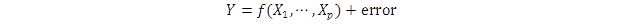Figure 5. Equation. Nonparametric regression model.

where, as usual, the error is assumed to be approximately normally distributed with mean 0 and unknown but constant variance (this assumption can be relaxed).

Nonparametric regression is similar to nonlinear regression, except that nonlinear regression requires one to specify the form of the relationship (e.g., logistic, sinusoidal, etc.) in advance.

In the context of traffic safety, nonparametric regression greatly extends the flexibility of the modeling; nearly all multiple regression models in highway safety analysis could be improved by the use of this tool. And nonparametric regression can extend the scope of application in unexpected ways. For example, the Cox proportional hazards model assumes that the roadway characteristics in the exponential function act as a linear regression, but nonparametric regression allows the generalization of the hazard function to the following: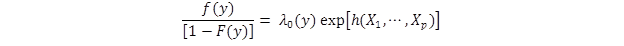Figure 6. Equation. Nonparametric regression model with a generalization of the hazard function.

where the h(.) function is a nonparametric regression. There are a number of nonparametric regression techniques that are available; a paper summarized in appendix D describes the use of Multivariate Adaptive Regression Splines to study red-light running, but one can also use Random Forests, Support Vector Machines, and other methods.

Appendix D also provides two recent and relevant examples on the use of non-parametric regression in traffic safety research.

### Principal Component Regression

Principal components analysis is used in multivariate analysis. For example, suppose one measured many things about someone's driving, such as average highway speed, maximum speed, the average highway following distance, average gap acceptance, and so forth. With a large sample of people, one might use principal components analysis to understand the correlation structure in the data. For example, it might be that the first principal axis is associated with speed, so that maximum speed and average speed load heavily on that axis. This first axis is the direction in the data space that explains the largest amount of the observed variation in the data. The next axis is perpendicular to the first, and might correspond to use of turn signals. It is the direction which is orthogonal to the first axis and which explains the largest proportion of the remaining variation. One can continue in this way, until one accounts for all the variation.

One could use the scores on each of these axes as explanatory variables for regression analysis, but the principal components that describe the data may not be the best ones for predicting the outcome of interest. In this example, the components that are listed might be good for predicting the probability that the driver will have an accident (after logit transformation to handle the fact that probabilities lie between 0 and 1), but the components listed would do a poor job of predicting how many miles the person drives in a day.

Principal components regression generalizes principal components analysis by finding the set of mutually perpendicular (orthogonal) axes such that the scores on those axes provide the strongest linear relationship (i.e., correlation) with the response variable of interest. In general, changing the response variable will lead to a different set of principal components. Since traffic safety studies often examine different responses, principal components regression could be helpful. It is closely related to partial least squares methods, and there are nonparametric generalizations, too.

### Hierarchical Bayesian Modeling

Hierarchical Bayes methods, which have been used in safety research, allow analysts to borrow information across similar but not identical situations, and to shrink estimates within a natural mathematical framework, which provably improves predictive accuracy. Borrowing can occur across outcome measures or predictor variables.

A hierarchical Bayesian model places distributions on the parameters used at lower levels in the model. For example, in a recent analysis of National Highway Transportation Safety Administration Fatality Analysis Reporting System data, a Poisson model was used for the number of accidents in a State, where the Poisson parameter was a linear combination of various predictors: unemployment rate, graduated license programs, age and state indicator variables, and so forth.(2) The regression coefficient on each of these was a hyperparameter, and thus had its own distribution. For example, the State effect was modeled as a random variable that was 1 of 50 draws from a normal distribution with unknown mean and large variance.

One result of this kind of model is that information from all 50 States can inform the estimates for each other. If Idaho has an unlucky year in terms of fatalities, the Idaho effect is still modeled as a draw from a normal distribution common to all States; thus, although the traditional estimate for unlucky Idaho would be very high, the other States are lower and this will pull the estimate from Idaho down from its unrepresentative high value. We say that the estimate for Idaho has "borrowed strength" from the data on the other States, and this has "shrunk" the estimate towards the common mean of all 50 States.

Appendix D summarizes one recent example from road safety research in which CMFs were developed from SPFs estimated with hierarchical Bayesian modeling.

### Spatial Kernel Averaged Predictors

Traditional spatial regression uses the measurements at a specific location to predict the response at that location. Spatial kernel averaging extends this by also using measurements from nearby locations.

For example, suppose one wanted to predict the number of accidents at a given intersection. One could use traffic volume, average speed, road type and signage at that location as predictors. But spatial kernel averaging would also include traffic volume data from nearby roads, average speed from nearby roads, and so forth. The weight on those other predictors would diminish as the distance from the location of interest increases. In some cases this may improve predictive accuracy by taking better account of large-scale factors such as shopping mall locations and local driving temperament. It is notable that the analyst does not have to specify these large-scale factors; the kernel averaging handles those effects automatically.

One advantage of this approach is that distance does not have to be geographic. It may be decided that intersections with similar features are "close" even if they are thousands of miles away from each other. Expert judgment may be used to determine the distance metric.

Appendix D provides a recent example from road safety research in which kernel averaging was used for developing safety performance functions for traffic analysis zones.

### Changepoint Detection

There are frequentist and Bayesian methods for doing changepoint detection, and it would make sense to use these methods to routinely monitor local crash rates to see if there are emerging problems. Such methods could help understand the impact of changes such as graduated licensure programs and drunk driving crackdowns, as well as flagging unexpected changes, which upon further examination, may be traced to changing populations or lax enforcement. For example, a changepoint that reflects improved safety appears in 2005, when the number of fatal crashes dropped precipitously, and the causal mechanisms should be studied to ensure that the improvement continues.

Changepoint methodology can also be helpful in identifying break points when creating "buckets," e.g., ranges of traffic volume for which an outcome measure, such as crashes, is fairly constant. Creating buckets is not always advisable, but it is often done in transportation science so as to increase the available sample size when studying rare events. The "cutpoints" that are relevant to traffic safety may be very different from the cutpoints that relate to fuel efficiency, and changepoint analysis offers a principled way to determine appropriate brackets. As such, it would be very useful for the disaggregate analysis that is usually undertaken in evaluations conducted for FHWA's Evaluation of Low Cost Countermeasures (ELCS) project. In particular, the methodology would be useful in establishing categorical variables that would be necessary for the development of CMFunctions that are seen as key to improving the transferability of future CMFs. For example, the methodology may be considered in National Cooperative Highway Research Program (NCHRP) Project 17-63, which is developing guidance for future researchers for the estimation of such CMFunctions.(3)

Changepoint methods can be used to find regions (buckets) in which the CMFunctions are locally linear or locally constant. That enables easier modeling and probably more accurate uncertainty statements when estimating the impact of specific safety measures.

Changepoint analysis is usually used to discover when a process has shifted or drifted away from its historical mean. In traffic safety, there was a huge drift down in fatalities that started in 2005. The usual approach for discovering when a shift/drift starts is to build a model for the historical process, a model for the change, and treat the changepoint time as a parameter to be estimated. Changepoint methodology is mature, and there are many sophisticated implementations that can be chosen to fit the specific application.

### Time Series Models and Distributed Lags

Distributed lag models are used in time series, where a future value is predicted as a linear regression upon previous values at different times. If, for example, one is trying to predict the number of vehicles on the road tomorrow, one might use a regression function that includes the amount of traffic today, the amount of traffic a week earlier than tomorrow, and the amount of traffic one year ago. This enables one to capture current effects (e.g., snowfall), weekend or weekday effects, and seasonal effects, respectively.

In transportation applications, there is the potential for more sophisticated applications than standard univariate time series. First, one can use dynamic factor models, in which latent factors determine the behavior. For example, it may be that the time series of hourly average traffic speed depends upon the mix of the drivers, so that the proportion of commuters, shoppers, and professional drivers on the road is an unobserved latent factor that affects the time series. Second, one could study multivariate time series, where one tracks over time two or more variables, say traffic speed and traffic volume, and these are correlated. Third, one could develop a new methodology for time series analysis when the unit of observation is network-valued, say the flows between specific points.

These models can become sophisticated and difficult. They should be considered carefully before adoption, since if the chosen model is not a good approximation to the process that generates the data, one can be misled. These models generally make strong assumptions and can be sensitive to small violations of those assumptions.

### Multivariate Nonparametric Tests

Rosenbaum proposed a nonparametric test for whether two multivariate populations are the same.(4) For example, suppose one wanted to decide whether there are differences in driving styles between people from Canada and the United States. One would then collect a random sample of 100 Canadian and 100 U.S. drivers and measure many features of their driving, such as following distance, maximum speed on highway, average speed on residential roads, etc. The next step is to build a metric: the distance between two people in the combined samples might be the Euclidean distance between their vectors of measurements, or it could be a more complicated distance that weights some features more heavily than others. Then one goes through all 200 people and finds the driver who is closest to each person in terms of this metric.

Under the null hypothesis that there is no difference between U.S. and Canadian drivers, it is equally likely that the nearest neighbor will be a U.S. or Canadian driver. Under the alternative hypothesis, U.S. drivers will tend to be neighbors of other U.S. drivers, and Canadian drivers will be neighbors of other Canadians. And the probabilities of a given number of same-same links are easy to calculate.

A good feature of this test is that one can try many different metrics, to explore which factors most distinctively separate U.S. and Canadian drivers. And, because of the combinatorial explosion in the number of possible links, the alpha level of the test is not as quickly eroded under multiple testing as usually happens.

### Other Methods

Statistics is rich in methodology, and some of it will apply to transportation safety. Currently, transportation scientists make heavy use of statistics, but their toolkit is based upon historical practice and may not be as current or as broad as is possible. Stronger collaborations with research statisticians will surely update the methodologies and promote better outcomes.